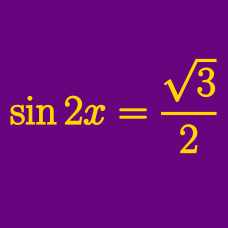Geometry

# Specific Solution of Simple Trigonometric Equations

How many solutions are there to $$\sin \theta + 7 = 8$$ in the domain $$[0 ^ \circ, 1000 ^ \circ ]$$?

What is the minimum value of a positive number $$x$$ such that the value of $$\sin(x+\frac{\pi}{8})$$ is $$0?$$

How many $$x$$'s in the interval $$0 \leq x \leq 15\pi$$ satisfy $$\tan x=-\sqrt{3}$$?

What are the solutions to $$12\sin \left( 2x-\frac{7}{6}\pi \right)=6\sqrt{2}$$ in the interval $$x \in [0,\pi]?$$

Which of the following is a solution to

$\sin ( 2 \theta - 31 ^ \circ ) = \cos 49 ^ \circ ?$

×

Problem Loading...

Note Loading...

Set Loading...